# Do Higher Prices Impact Smoking? Estimating the Elasticity of Demand of Cigarettes Using 2 Stage-Least-Squares (2SLS)

Posted 2018-10-16

## I. Motivating Question*:¶

What is the demand curve look like for cigarettes? Is demand sensitive to prices? If so, taxes are potential tools to lower demand.

But how do we estimate the demand curve when we just observe data and prices are determined by the intersection of both supply and demand curves?

Most of the technical analysis is reproduced from the following:

Stock and Watson 2015 - Introduction to Econometrics Ch 12. Instrumental Variables Regression

William A. Sundstrom - Guide to R For SCU Economics Students - Instrumental Variables Regression

Fiorentini, Gabriele - Econometrics Course, Lesson 10

#### Intro:¶

Regardless whether we are smokers or non-smokers, as a society we've taken on many efforts to curb demand of cigarettes. So understanding the tools that impact demand seems useful.

Select events from the chronology documented at National Institute of Health

• 1957 --Surgeon General Leroy E. Burney (1956-1961) declared it to be the official position of the U.S. Public Health Service that a causal relationship exists between smoking and lung cancer
• 1964 --Surgeon General Luther L. Terry (1961-65) issued Smoking and Health, the first Surgeon General's report to receive widespread media and public attention link to report
• 1965 --Congress mandated health warnings on cigarette packs
• 1969 --The Public Health Cigarette Smoking Act passed in Congress. It imposed a ban on cigarette advertising on television and radio after September 30, 1970, and required that the Surgeon General produce an annual report on the latest scientific findings on the health effects of smoking
• 1983 --Lung cancer surpassed breast cancer as the leading cause of death from cancer in women

Other literature modeling the effects of price and smoking prevalence

Chaloupka FJ, Yurekli A, Fong GT, Tobacco taxes as a tobacco control strategy, Tobacco Control 2012;21:172-180. https://tobaccocontrol.bmj.com/content/21/2/172

Frank J. Chaloupka, IV, Richard Peck, John A. Tauras, Xin Xu, Ayda Yurekli, Cigarette Excise Taxation: The Impact of Tax Structure on Prices, Revenues, and Cigarette Smoking, 2010, NBER Working Paper No. 16287 http://www.nber.org/papers/w16287

WHO (2010), Technical Manual on Tobacco Tax Administration. World Health Organization, Geneva. http://www.who.int/tobacco/publications/tax_administration/en/ http://www.who.int/tobacco/publications/en_tfi_tob_tax_chapter2.pdf

## III. Approach¶

### Idealized Experiment vs Reality:¶

If everyone were given random prices and we could observe their cigarette demand at those prices. In aggregate we would be able to estimate the demand curve and corresponding elasticity.

Though we are not able to achieve this at the individual level, we have data at the state level for a particular year (1995)

It also needs to be noted that the price is typically set by both supply and demand, so this simultaneous causality creates a situation where the price is actually endogenous to demand, not independent of demand.

To tease this out, we need to implement an instrument variable of sales tax (which is decided outside of demand of cigarettes and prices) that will allows us to tease out the effect of change in price to change in demand, as this will allow us to trace along the demand curve by holding the demand curve fixed and moving the supply curve.

In doing so if we just did OLS, the elasticity estimator would be systematically bias (ie. not consistent).

### Data:¶

AER cigarettesSW dataset documentation* Note that the documentation does not say where the data comes from or how it's collected (so I just assume worst-case that it's for illustrative purposes, ie. not to treat it as actual data as input for other analysis)

• I exported the dataset from R to csv so I can import into pandas
• Panel data on cigarette consumption for the 48 continental US States from 1985--1995
• There isn't more details about the dataset, so I assume it to be aggregated admin data at the state level.
• The data frame contains 48 observations on 7 variables for 2 periods.

state: Factor indicating state.

year: Factor indicating year.

cpi: Consumer price index.

population: State population.

packs: Number of packs per capita.

income: State personal income (total, nominal).

tax: Average state, federal and average local excise taxes for fiscal year.

price: Average price during fiscal year, including sales tax.

taxs: Average excise taxes for fiscal year, including sales tax.

## IV. Organizing / Exploring the Data¶

In :
from linearmodels.iv import IV2SLS, IVGMM
import matplotlib.pyplot as plt
import numpy as np
import pandas as pd
from scipy import stats
import seaborn as sns
import statsmodels.api as sm

%matplotlib inline

In :
df_raw = pd.read_csv('./cigarettesSW.csv', index_col='index')
df_raw = df_raw[['population', 'packs', 'income', 'tax', 'price', 'taxs', 'year', 'cpi']]

Out:
population packs income tax price taxs year cpi
index
1 3973000.0 116.486282 46014968 32.500004 102.181671 33.348335 1985 1.076
2 2327000.0 128.534592 26210736 37.000000 101.474998 37.000000 1985 1.076
3 3184000.0 104.522614 43956936 31.000000 108.578751 36.170418 1985 1.076
4 26444000.0 100.363037 447102816 26.000000 107.837341 32.104000 1985 1.076
5 3209000.0 112.963539 49466672 31.000000 94.266663 31.000000 1985 1.076
In :
for _ in df_raw.columns:
print(_, df_raw[_].dtype)

population float64
packs float64
income int64
tax float64
price float64
taxs float64
year int64
cpi float64


### Compare raw data 1985 vs 1995¶

In :
def summary_plot_2periods(boxplot, colnames):
fig, axes = plt.subplots(len(colnames) // 2, 2, figsize=(12, 8))
k = 0
for i in range(len(colnames) // 2):
for j in range(2):
if boxplot:
df_raw.boxplot(column=colnames[k], by='year', ax=axes[(i, j)])
else:
sns.violinplot('year', colnames[k], data=df_raw, ax=axes[(i, j)])
k += 1

In :
colnames = ['population', 'packs', 'income', 'tax', 'price', 'taxs']
summary_plot_2periods(boxplot=True, colnames=colnames)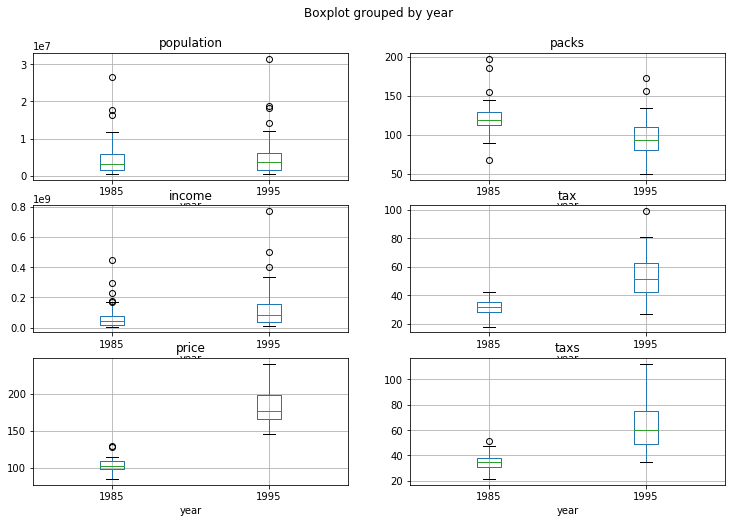In :
summary_plot_2periods(boxplot=False, colnames=colnames)

/anaconda3/envs/aind-dl/lib/python3.5/site-packages/scipy/stats/stats.py:1713: FutureWarning: Using a non-tuple sequence for multidimensional indexing is deprecated; use arr[tuple(seq)] instead of arr[seq]. In the future this will be interpreted as an array index, arr[np.array(seq)], which will result either in an error or a different result.
return np.add.reduce(sorted[indexer] * weights, axis=axis) / sumval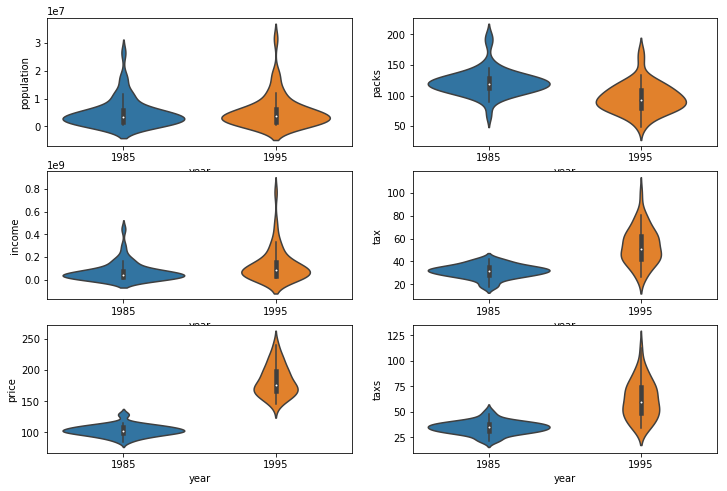#### Observations:¶

• Violinplots are kernel density estimates so funkiness can occur in smoothing. Here we can see population dipping into negatives, which obviously doesn't make sense.
• Big increase in prices and taxes from 1985 -> 1995.
• Notable decrease in packs from 1985 -> 1995.

## V. Model Specification of the Demand Curve¶

$$\ln Packs_{1995} = \beta_0 + \beta_1 \ln Price_{1995} + u_{1995} \tag{1}$$

where $\beta_1$ is the parameter of interest since that is $elasticity \, \epsilon$

Price Elasticity of Cigarettes Supply and demand curve - endogenous

$$elasticity\, \epsilon = \frac{\Delta Quantity}{\Delta Price}$$

### Features Engineering¶

In :
# Return copy of df to ensure no SettingWithCopyWarnings
df = df_raw.copy()

# Transform by taking logs and correcting for CPI
df['ln_packs_per_cap'] = np.log(df_raw['packs'])
df['ln_avg_price'] = np.log(df_raw['price'] / df_raw['cpi'])
df['ln_income_per_cap'] = np.log(df_raw['income'] / df_raw['population'] / df_raw['cpi'])
df['sales_tax'] = (df_raw['taxs'] - df_raw['tax']) / df_raw['cpi']
df['cig_tax'] = df_raw['tax'] / df_raw['cpi']

df_1995 = df[df['year'] == 1995]
df_1985 = df[df['year'] == 1985]

In :
fig, ax = plt.subplots()
ax.scatter(x = (df_1995['price'] / df_1995['cpi']), y = df_1995['packs'], label = '1995')
ax.scatter(x = (df_1985['price'] / df_1985['cpi']), y = df_1985['packs'], label = '1985')
ax.legend()
ax.set_xlabel('Avg price during fiscal year, incl sales tax')
ax.set_ylabel('Number of packs per capita')
ax.set_title('# Packs vs Price by year')

Out:
<matplotlib.text.Text at 0x1c1a13ff28>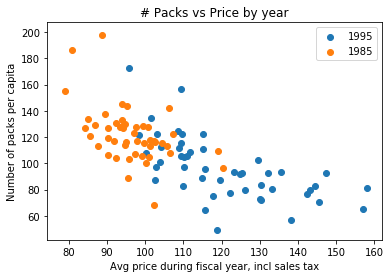#### Observations:¶

• Definitely a downward sloping trend as price increase. Similar to what we'd expect for a demand curve.
In :
fig, ax = plt.subplots()
ax.scatter(x = (df_1995['ln_avg_price']), y = df_1995['ln_packs_per_cap'], label = '1995')
ax.legend()
ax.set_xlabel('ln avg price')
ax.set_ylabel('ln packs per cap')
ax.set_title("ln # Packs vs ln Price in 1995")

Out:
<matplotlib.text.Text at 0x1c1a965f98>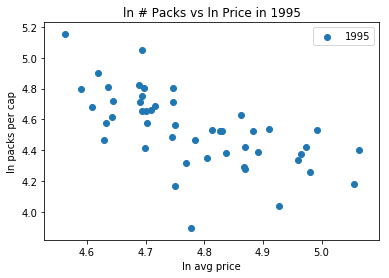#### Observations:¶

• By taking the log of both price and packs, the linear negative trend for 1995 is clearer.
In :
fig, ax = plt.subplots()
ax.scatter(x = (df_1995['sales_tax'] / (df_1995['price'] - df_1995['taxs'])),
y = df_1995['cig_tax'] / (df_1995['price'] - df_1995['taxs']), label = '1995')
ax.scatter(x = (df_1985['sales_tax'] / (df_1985['price'] - df_1985['taxs'])),
y = df_1985['cig_tax'] / (df_1985['price'] - df_1985['taxs']), label = '1985')
ax.legend()
ax.set_xlabel('% sales tax')
ax.set_ylabel('% cig tax')
ax.set_title('% Cigarette Tax vs Sales Tax by Year')

Out:
<matplotlib.text.Text at 0x1c1aaaecc0>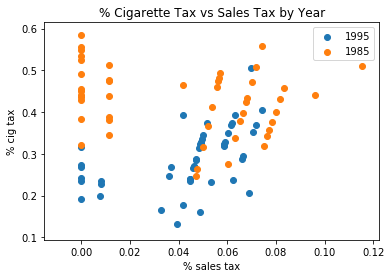### Model selection 1: Ordinary Least Squares (OLS)¶

In :
OLS_model = sm.OLS.from_formula('ln_packs_per_cap ~ ln_avg_price + 1', df_1995).fit()
b0, b1 = OLS_model.params
print(OLS_model.summary())

                            OLS Regression Results
==============================================================================
Dep. Variable:       ln_packs_per_cap   R-squared:                       0.406
Method:                 Least Squares   F-statistic:                     31.41
Date:                Fri, 26 Oct 2018   Prob (F-statistic):           1.13e-06
Time:                        19:29:32   Log-Likelihood:                 12.724
No. Observations:                  48   AIC:                            -21.45
Df Residuals:                      46   BIC:                            -17.71
Df Model:                           1
Covariance Type:            nonrobust
================================================================================
coef    std err          t      P>|t|      [0.025      0.975]
--------------------------------------------------------------------------------
Intercept       10.3389      1.035      9.986      0.000       8.255      12.423
ln_avg_price    -1.2131      0.216     -5.604      0.000      -1.649      -0.777
==============================================================================
Omnibus:                       11.094   Durbin-Watson:                   1.985
Prob(Omnibus):                  0.004   Jarque-Bera (JB):               12.802
Skew:                          -0.823   Prob(JB):                      0.00166
Kurtosis:                       4.921   Cond. No.                         189.
==============================================================================

Warnings:
 Standard Errors assume that the covariance matrix of the errors is correctly specified.

In :
fig, ax = plt.subplots()
ax.scatter(df_1995['ln_avg_price'], df_1995['ln_packs_per_cap'], label = '1995')
ax.plot(df_1995['ln_avg_price'], b0 + b1 * df_1995['ln_avg_price'], color = 'orange', lw = 1, label = 'OLS model')
ax.legend()
ax.set_xlabel('ln avg price')
ax.set_ylabel('ln packs per cap')
ax.set_title('OLS Model')

Out:
<matplotlib.text.Text at 0x1c1ab52dd8>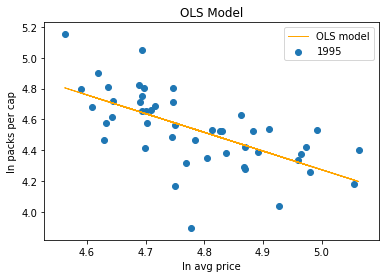In :
plt.scatter(OLS_model.fittedvalues, OLS_model.resid)
plt.axhline(0, linestyle=':', color='orange')
plt.title('OLS Model Residuals')

Out:
<matplotlib.text.Text at 0x1c19edce10>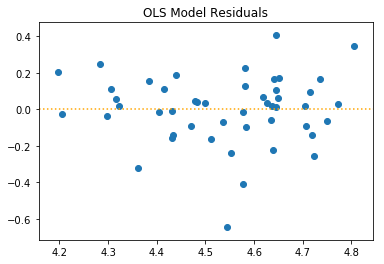#### OLS Results:¶

• It's clear from the plot that there is a negative trend (increasing price -> decreasing # of packs per cap)
• $\beta_1$ for ln_avg_price -1.21 (SE 0.22) and t-stat -5.6
• Intuitively this means 1% increase in price means -1.21%
• Residuals seem to have a little bit of a V shaped pattern

#### When can we generally trust OLS?¶

• Linearity
• Given 2 random variables X, Y and residual (ie. error) U -> $Y = E[Y|X] + U$ we impose linearity so it is $Y = \beta_0 + \beta_1 X + U$
• Expected value of the residuals is 0 -> $E[U|X] = 0$. ie. mean of the errors is 0, but doesn't mean it has to be symmetric
• Simple random sample
• Random variables X and Y are independent and identically distributed (IID) - ie. independent draws from their joint distribution
• No extreme outliers
• $0 < E[X^4] < \infty$ and $0 < E[U^4] < \infty$
• Consistency so that the estimators converge to their true value (which is generally unknown)
• No perfect collinearity
• No exact linear relationship between the independent variables (ie. multiples or linear combination)

#### Issues?¶

• Is price really exogenous to quantity of cigarettes? No, since price will indeed affect the quanity but quanity will also affect price (since there is actually 2 curves: supply and demand). Y and X flows both ways and X is no longer an independent variable but now endogenous.
• Simultaneous causality - as price increases demand decreases. As demand decreases, price increases (ie. the 2 interact).
• Have we omitted any variables? If so, that'll be lumped in with U which means $E[U|X]$ will no longer = 0

### Model selection 2: 2 Stage Least Squares (2SLS) / Instrument Variable Regression (IV)¶

#### Instrument variables and endogeneity:¶

Because price is an endogenous variable (given the interactions of supply and demand curve), we use an instrument variable Z to help us. One that we know will not move the demand curve and trace the demand curve through shifts in supply curve.

Z needs to satisfy these 3 conditions:

• Relevance to price -> $cov(Z, X) \neq 0$, ie, a predictor for P which means non-zero slope in OLS regression for price (generally easier to satisfy)
• Exogenous -> $cov(Z, U) = 0$, ie. not correlated with error u (generally hard to satisfy)
• Exclusion restriction - effect on Q only thru P and not on Q directly
• Z is exogenous (ie. determined outside the system and does not influence or correlate with demand by itself)

#### 2SLS - Stage 1 OLS:¶

##### Assumption 1 Relevance: Regress endogeneous variable with potential IV¶
$$x_i = \pi_0 + \pi_1 Z_i + v_i \tag 2$$$$\widehat{\ln Price_{1995}} = \pi_0 + \pi_1 IV_{1995} + v_{1995} \tag 3$$

In :
matrix_vars = ['ln_packs_per_cap', 'ln_avg_price', 'ln_income_per_cap', 'sales_tax', 'cig_tax']
n_vars = len(matrix_vars)
z_score = 1.98
pair = {}

fig, axes = plt.subplots(n_vars, n_vars, figsize=(30, 30))
for m, row_idx in zip(matrix_vars, range(n_vars)):
for n, col_idx in zip(matrix_vars, range(n_vars)):
if m != n:
param = {}
axes[row_idx, col_idx].set_xlabel(m)
axes[row_idx, col_idx].set_ylabel(n)
axes[row_idx, col_idx].scatter(df_1995[m], df_1995[n])
#slope, intercept, r_value, p_value, std_err = stats.linregress(x = df_1995[m], y = df_1995[n])
param['slope'], param['intercept'], param['r_value'], param['p_value'], param['std_err'] = stats.linregress(x = df_1995[m], y = df_1995[n])
ci_lb, ci_ub = sorted([param['slope'] - z_score * param['std_err'], param['slope'] + z_score * param['std_err']])
if ci_ub * ci_lb > 0:
color = 'red'
else:
color = 'black'
axes[row_idx, col_idx].plot(df_1995[m], param['slope'] * df_1995[m] + param['intercept'], color = color)
axes[row_idx, col_idx].set_title("b0: {3:.2f}, b1: {2:.2f}, r: {4:.2f} 95%:({0:.2f}, {1:.2f})"
.format(ci_lb, ci_ub, param['slope'], param['intercept'], param['r_value']))
pair[n, m] = param
else:
continue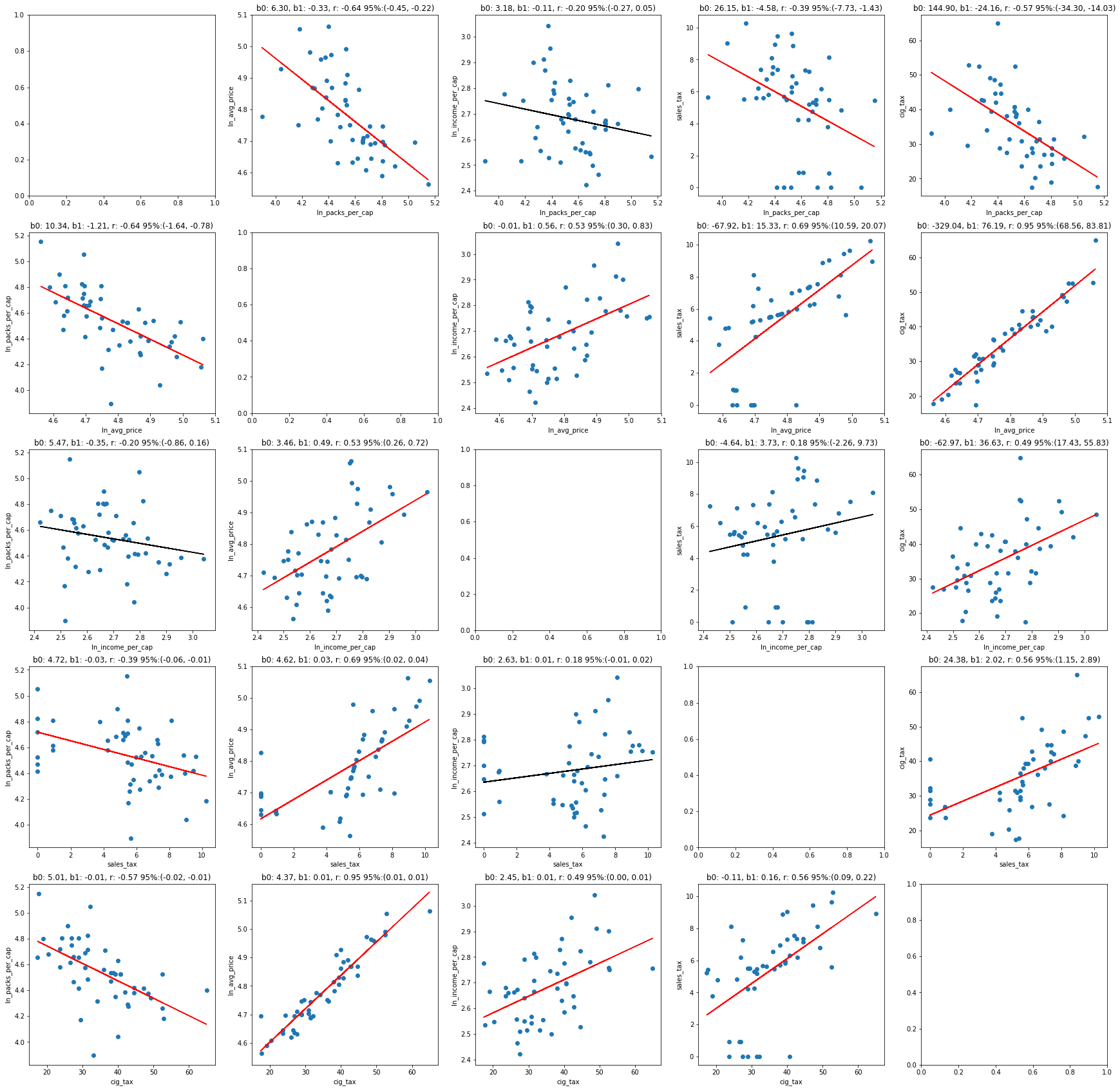In :
potential_IV = ['ln_income_per_cap', 'sales_tax', 'cig_tax']
for var in potential_IV:
print(var, 'slope: {0:.4f}\t\t p_value: {1:.4f}'.format(pair['ln_avg_price', var]['slope'],
pair['ln_avg_price', var]['p_value']))

ln_income_per_cap slope: 0.4915		 p_value: 0.0001
sales_tax slope: 0.0307		 p_value: 0.0000
cig_tax slope: 0.0117		 p_value: 0.0000

$$\widehat{\ln Price_{1995}} = \pi_0 + \pi_1 Sales\_ Tax_{1995} + \pi_2 Cigarette\_ Tax_{1995} + v_{1995} \tag 4$$
In :
OLS_model = sm.OLS.from_formula('ln_avg_price ~ sales_tax + cig_tax', df_1995).fit()
print(OLS_model.summary())

                            OLS Regression Results
==============================================================================
Dep. Variable:           ln_avg_price   R-squared:                       0.930
Method:                 Least Squares   F-statistic:                     300.1
Date:                Fri, 26 Oct 2018   Prob (F-statistic):           9.55e-27
Time:                        19:29:37   Log-Likelihood:                 95.060
No. Observations:                  48   AIC:                            -184.1
Df Residuals:                      45   BIC:                            -178.5
Df Model:                           2
Covariance Type:            nonrobust
==============================================================================
coef    std err          t      P>|t|      [0.025      0.975]
------------------------------------------------------------------------------
Intercept      4.3687      0.018    243.664      0.000       4.333       4.405
sales_tax      0.0102      0.002      4.780      0.000       0.006       0.014
cig_tax        0.0102      0.001     17.213      0.000       0.009       0.011
==============================================================================
Omnibus:                        1.372   Durbin-Watson:                   1.552
Prob(Omnibus):                  0.504   Jarque-Bera (JB):                0.818
Skew:                           0.311   Prob(JB):                        0.664
Kurtosis:                       3.149   Cond. No.                         134.
==============================================================================

Warnings:
 Standard Errors assume that the covariance matrix of the errors is correctly specified.

$$\widehat{\ln Price_{1995}} = \pi_0 + \pi_1 Sales\_ Tax_{1995} + \pi_2 Cigarette\_ Tax_{1995} + \pi_3 \ln Income_{1995} + v_{1995} \tag 5$$
In :
OLS_model = sm.OLS.from_formula('ln_avg_price ~ sales_tax + cig_tax + ln_income_per_cap', df_1995).fit()
print(OLS_model.summary())

                            OLS Regression Results
==============================================================================
Dep. Variable:           ln_avg_price   R-squared:                       0.940
Method:                 Least Squares   F-statistic:                     231.1
Date:                Fri, 26 Oct 2018   Prob (F-statistic):           6.10e-27
Time:                        19:29:37   Log-Likelihood:                 98.806
No. Observations:                  48   AIC:                            -189.6
Df Residuals:                      44   BIC:                            -182.1
Df Model:                           3
Covariance Type:            nonrobust
=====================================================================================
coef    std err          t      P>|t|      [0.025      0.975]
-------------------------------------------------------------------------------------
Intercept             4.1030      0.099     41.492      0.000       3.904       4.302
sales_tax             0.0109      0.002      5.422      0.000       0.007       0.015
cig_tax               0.0094      0.001     14.909      0.000       0.008       0.011
ln_income_per_cap     0.1083      0.040      2.726      0.009       0.028       0.188
==============================================================================
Omnibus:                        0.060   Durbin-Watson:                   1.605
Prob(Omnibus):                  0.970   Jarque-Bera (JB):                0.259
Skew:                          -0.003   Prob(JB):                        0.878
Kurtosis:                       2.640   Cond. No.                         850.
==============================================================================

Warnings:
 Standard Errors assume that the covariance matrix of the errors is correctly specified.


#### Observations:¶

• ln_income_per_cap, sales_tax, cig_tax are all relevant for price
• Though combo of sales_tax is cig_tax seems best since ln_income_per_cap's t-stat is only 2.7 for the model in equation 5, we leave ln_income_per_cap in because the instruments sales_tax and cig_tax need to hold even in the prescence of ln_income_per_cap
• t-stat - neither sales_tax nor cig_tax are weak instruments (generally accepted cutoff is 4-5)
• sales_tax = 4.8
• cig_tax = 19.8
• f-stat - generally accepted cutoff is 15-20
• sales_tax = 40
• cig_tax = 391
• sales_tax and cig_tax = 300
• sales_tax and cig_tax and ln_income_per_cap = 231
• If they were weak instruments cov(price, sales_tax) or cov(price, cig_tax) would be tiny and estimator could be biased since they could blow up
##### Assumption 2 Exclusionary Restriction: IV must only affect Y thru endogenous X, not by itself¶
$$\ln Packs_{1995} = \ln Price_{1995} + potential \, IV_{1995}$$
In :
exclusion_model = {}
IV_df = pd.DataFrame()

print('Does IV have effect on Y by itself?')
for IV in potential_IV:
exclusion_model[IV] = sm.OLS.from_formula('ln_packs_per_cap ~ ln_avg_price + ' + IV, df_1995).fit()
print('\n-----', IV, '-----')
print('p-value: {0:.3f}'.format(exclusion_model[IV].pvalues[len(exclusion_model[IV].pvalues)-1]))

Does IV have effect on Y by itself?

----- ln_income_per_cap -----
p-value: 0.150

----- sales_tax -----
p-value: 0.578

----- cig_tax -----
p-value: 0.403


#### Observations:¶

• None of the potential instruments affect Y directly by itself
##### Assumption 3 Correlation with Error: Regress potential IV with error (from OLS model with sales tax as exogenous var? or from 2SLS?)¶

We will need to examine using J-test in the over-identified case (2 instruments and 1 endogenous var)

### Which instruments should we use?¶

In addition to fulfilling the 3 assumptions above, we need to argue that the IV is plausible.

State sales tax is a good candidate since it conceptually is exogenous to the system. A higher sales tax means higher prices but quantity does not dictate sales tax, as it is determined by state regulation for all goods. Sales tax also should not affect demand directly. In other words, smokers' generally do not care about whether there is a sales tax, only what the resulting price is (which state tax influences).

Cigarette tax likewise has the same attributes as sales tax, though maybe one can argue there is an indirect effect of lower demand would likely induce the state regulators to lower cigarette taxes.

### 2SLS Stage 2 with IV's¶

#### w/ IV Sales Tax¶

\eqalign{ \ln Packs_{1995} &= \beta_0 + \beta_1 \widehat {\ln Price_i} + u_i \tag 6 \\ \widehat{\ln Price_{1995}} &= \pi_0 + \pi_1 Sales\_ Tax_{1995} + v_{1995} }
In :
twoSLS_model = IV2SLS.from_formula('ln_packs_per_cap ~ [ln_avg_price ~ sales_tax] + 1', df_1995).fit()
print(twoSLS_model.summary)

                          IV-2SLS Estimation Summary
==============================================================================
Dep. Variable:       ln_packs_per_cap   R-squared:                      0.4011
No. Observations:                  48   F-statistic:                    12.046
Date:                Fri, Oct 26 2018   P-value (F-stat)                0.0005
Time:                        19:29:37   Distribution:                  chi2(1)
Cov. Estimator:                robust

Parameter Estimates
================================================================================
Parameter  Std. Err.     T-stat    P-value    Lower CI    Upper CI
--------------------------------------------------------------------------------
Intercept        9.7199     1.4961     6.4966     0.0000      6.7875      12.652
ln_avg_price    -1.0836     0.3122    -3.4708     0.0005     -1.6955     -0.4717
================================================================================

Endogenous: ln_avg_price
Instruments: sales_tax
Robust Covariance (Heteroskedastic)
Debiased: False


#### w/ IV Cigarette Tax¶

\eqalign{ \ln Packs_{1995} &= \beta_0 + \beta_1 \widehat {\ln Price_i} + u_i \tag {7} \\ \widehat{\ln Price_{1995}} &= \pi_0 + \pi_1 Cig\_ Tax_{1995} + v_{1995} }
In :
twoSLS_model = IV2SLS.from_formula('ln_packs_per_cap ~ [ln_avg_price ~ cig_tax] + 1', df_1995).fit()
print(twoSLS_model.summary)

                          IV-2SLS Estimation Summary
==============================================================================
Dep. Variable:       ln_packs_per_cap   R-squared:                      0.4047
No. Observations:                  48   F-statistic:                    35.849
Date:                Fri, Oct 26 2018   P-value (F-stat)                0.0000
Time:                        19:29:37   Distribution:                  chi2(1)
Cov. Estimator:                robust

Parameter Estimates
================================================================================
Parameter  Std. Err.     T-stat    P-value    Lower CI    Upper CI
--------------------------------------------------------------------------------
Intercept        10.039     0.9255     10.847     0.0000      8.2246      11.852
ln_avg_price    -1.1502     0.1921    -5.9874     0.0000     -1.5267     -0.7737
================================================================================

Endogenous: ln_avg_price
Instruments: cig_tax
Robust Covariance (Heteroskedastic)
Debiased: False


#### w/ IV Cigarette Tax and Sales Tax¶

\eqalign{ \ln Packs_{1995} &= \beta_0 + \beta_1 \widehat {\ln Price_i} + \ln Income + u_i \tag 8 \\ \widehat{\ln Price_{1995}} &= \pi_0 + \pi_1 Sales\_ Tax_{1995} + \pi_2 Cig\_ Tax_{1995} + v_{1995} }
In :
twoSLS_model = IV2SLS.from_formula('ln_packs_per_cap ~ [ln_avg_price ~ sales_tax + cig_tax] + ln_income_per_cap + 1',
df_1995).fit()
print(twoSLS_model.summary)

                          IV-2SLS Estimation Summary
==============================================================================
Dep. Variable:       ln_packs_per_cap   R-squared:                      0.4294
No. Observations:                  48   F-statistic:                    34.506
Date:                Fri, Oct 26 2018   P-value (F-stat)                0.0000
Time:                        19:29:37   Distribution:                  chi2(2)
Cov. Estimator:                robust

Parameter Estimates
=====================================================================================
Parameter  Std. Err.     T-stat    P-value    Lower CI    Upper CI
-------------------------------------------------------------------------------------
Intercept             9.8950     0.9288     10.654     0.0000      8.0746      11.715
ln_income_per_cap     0.2804     0.2458     1.1407     0.2540     -0.2014      0.7622
ln_avg_price         -1.2774     0.2417    -5.2855     0.0000     -1.7511     -0.8037
=====================================================================================

Endogenous: ln_avg_price
Instruments: sales_tax, cig_tax
Robust Covariance (Heteroskedastic)
Debiased: False

In :
plt.scatter(twoSLS_model.fitted_values, twoSLS_model.resids)
plt.title('2SLS w/ sales_tax and cig_tax residuals')

Out:
<matplotlib.text.Text at 0x1c1ca43c18>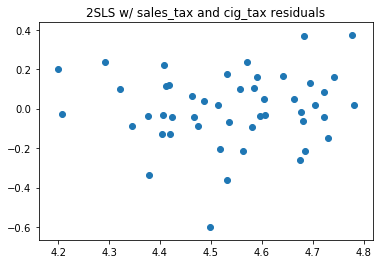#### Checking if the instruments exogenous when overidentified - 2 IVs and 1 endogenous var¶

In :
GMM_model = IVGMM.from_formula('ln_packs_per_cap ~ [ln_avg_price ~ sales_tax + cig_tax] + ln_income_per_cap + 1',
df_1995).fit()
print(GMM_model.summary)

                          IV-GMM Estimation Summary
==============================================================================
Dep. Variable:       ln_packs_per_cap   R-squared:                      0.4302
No. Observations:                  48   F-statistic:                    34.467
Date:                Fri, Oct 26 2018   P-value (F-stat)                0.0000
Time:                        19:29:38   Distribution:                  chi2(2)
Cov. Estimator:                robust

Parameter Estimates
=====================================================================================
Parameter  Std. Err.     T-stat    P-value    Lower CI    Upper CI
-------------------------------------------------------------------------------------
Intercept             9.8961     0.9346     10.588     0.0000      8.0642      11.728
ln_income_per_cap     0.3179     0.2378     1.3369     0.1813     -0.1481      0.7839
ln_avg_price         -1.2987     0.2401    -5.4084     0.0000     -1.7694     -0.8281
=====================================================================================

Endogenous: ln_avg_price
Instruments: sales_tax, cig_tax
GMM Covariance
Debiased: False
Robust (Heteroskedastic)

In :
GMM_model.j_stat

Out:
H0: Expected moment conditions are equal to 0
Statistic: 0.3347
P-value: 0.5629
Distributed: chi2(1)
WaldTestStatistic, id: 0x1c1cb67a90

#### Observations¶

• Since we have 2 IV's, it is overidentified, therefore we can run the 2SLS with each IV separately and see if the estimators are similar
• sales_tax only: -1.08 (SE 0.31)
• cig_tax only: -1.15 (SE 0.19)
• cig_tax & sales_tax: -1.28 (SE 0.24)
• As noted before we still need to include ln_income_per_cap even though it's not technically signifcant as it'll impact elasticity coefficient and instruments must still hold in light of its presence.
• J stat is not significant so we cannot reject the null. Thus the 2 instruments seem to be exogenous

## VI. Results¶

#### OLS vs 2SLS Results¶

$OLS \, elasticity \, \epsilon = -1.21 (SE 0.22) \tag {results (eq. 1)}$

$2SLS \, elasticity \, \epsilon = -1.28 (SE 0.24) \tag {results (eq. 8)}$

F-stat of stage 1 and 2 of 2SLS with the 2 IV's all pass the thresholds.

In this case, the OLS model doesn't seem significantly biased given the estimators from the 2 models are not statistically different from each other. Regardless, the 2SLS model with instrument variables (sales tax and cigarette tax) is the approach approach to deal with simultaneous causality issues.

Intutitvely this model implies that a 1% increase in price will correspond to a -1.28% decrease in packs per capita, but only within this range that we have data for (ie. if we double the price, it does not mean demand will go to 0). This is a suprisingly high elasticity.

In addition, this model also indicated low income elasticity, ie. the income/capita doesn't affect demand (see appendix for analysis).

## Appendix¶

### For 1985 for reference (we can potentially run pooled with 1995 and set year as dummy variable to leverage more data or look at short term vs long term elasticity)¶

$$\ln Packs_{1985} = \beta_0 + \beta_1 \widehat {\ln Price_{1985}} + u_{1985} \tag {A. 2}$$
In :
twoSLS_model = IV2SLS.from_formula('ln_packs_per_cap ~ [ln_avg_price ~ sales_tax + cig_tax] + ln_income_per_cap + 1',
df_1985).fit()
print(twoSLS_model.summary)

                          IV-2SLS Estimation Summary
==============================================================================
Dep. Variable:       ln_packs_per_cap   R-squared:                      0.2964
No. Observations:                  48   F-statistic:                    18.920
Date:                Fri, Oct 26 2018   P-value (F-stat)                0.0001
Time:                        19:29:38   Distribution:                  chi2(2)
Cov. Estimator:                robust

Parameter Estimates
=====================================================================================
Parameter  Std. Err.     T-stat    P-value    Lower CI    Upper CI
-------------------------------------------------------------------------------------
Intercept             8.5101     0.9189     9.2607     0.0000      6.7090      10.311
ln_income_per_cap     0.2769     0.1700     1.6295     0.1032     -0.0562      0.6100
ln_avg_price         -0.9696     0.2237    -4.3335     0.0000     -1.4081     -0.5310
=====================================================================================

Endogenous: ln_avg_price
Instruments: sales_tax, cig_tax
Robust Covariance (Heteroskedastic)
Debiased: False